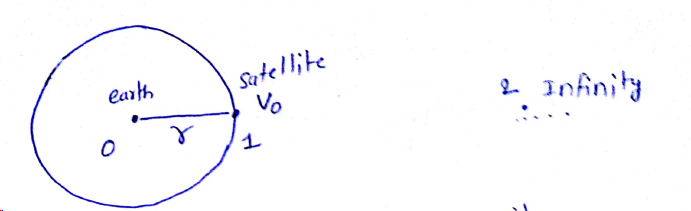# A stellite is moving with a constant speed  v in circular orbit around the earth. An object of mass 'm' is ejected from the satellite such that it just escapes from the gravitational pull of the earth. At the time of ejection, the kinetic energy of the object is:Option 1)Option 2)Option 3)Option 4)Relation of escape velocity and orbital velocity -

Orbital velocity

Escape velocity

- whereinorbital velocity .

Apply energy conservation for point 1 and point 2 .

for satellite to escape to infinity

we take (at infinity       PE=KE=0)

so

Option 1)Option 2)Option 3)Option 4)Exams
Articles
Questions